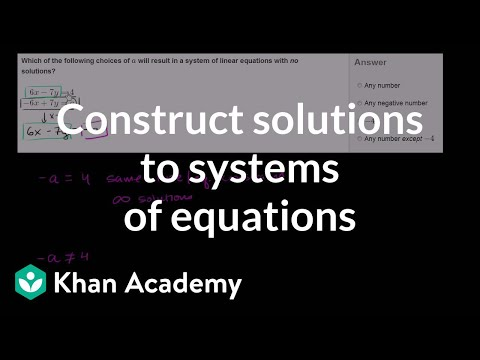Video

# Systems of equations with graphing (Full video)

Khan Academy

## Supporting grades: 8

Description: Sal shows how to solve a system of linear equations by graphing and looking for the point of intersection. Created by Sal Khan. Let's say I have the equation y is equal to x plus 3. And I want to graph all of the sets, all of the coordinates x comma y that satisfy this equation right there. So this line is going to look like this.

You must log inorsign upif you want to:*

*Teacher Advisor is 100% free.

### Other videos you might be interested in### Completing solutions to 2-variable equations (Full video)

#### Khan Academy